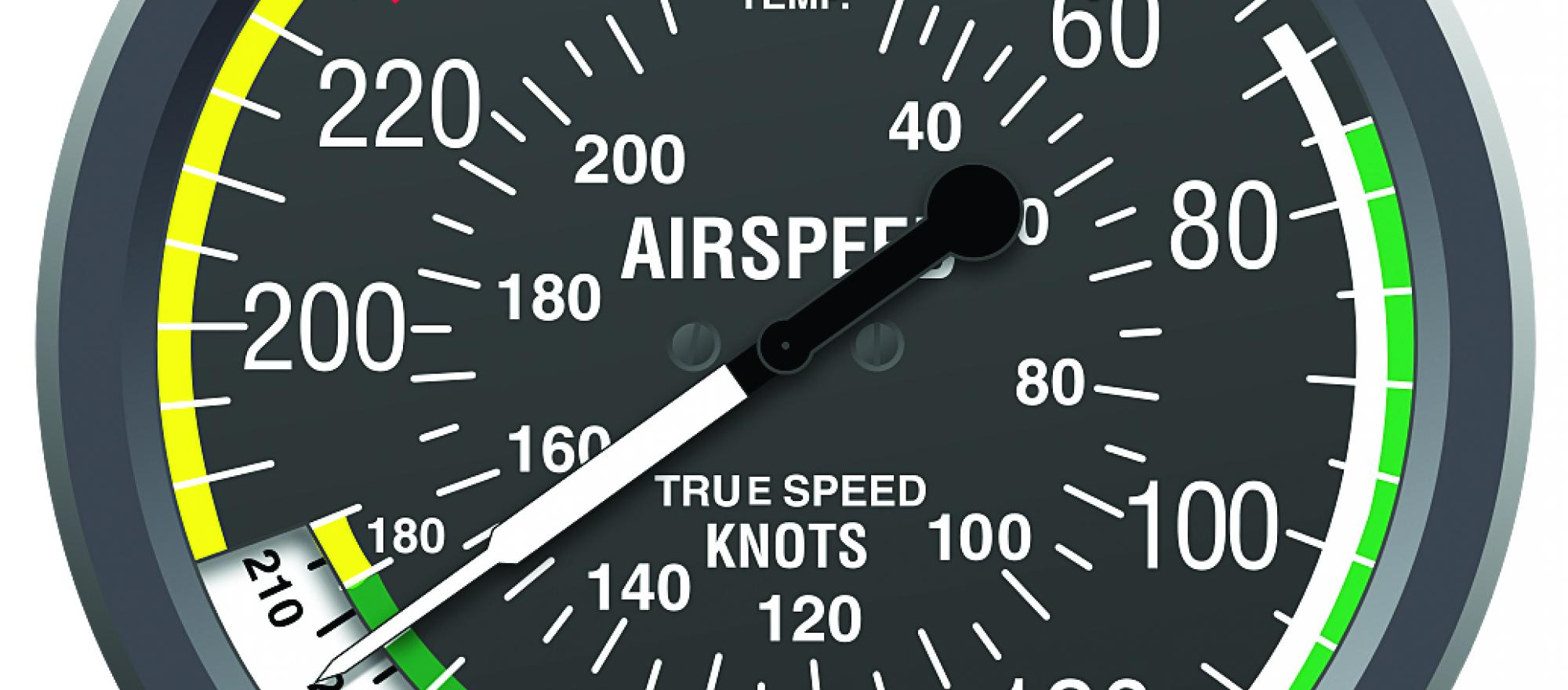Select PageAt mean sea level, with all conditions being the same, indicated airspeed (IAS) will the same as true airspeed (TAS). However, when the aircraft gains in altitude, or the temperature increases (causing the air density to decrease), then surprisingly, the TAS ‘increases’. Before I get into explaining this, let me first remind you why we need to know what the TAS is. True airspeed is the calibrated airspeed (CAS) corrected for altitude and non-standard temperature, which means that it needs to be calculated. The assumption here is that the aircraft that we are flying is slow (less than 250kts), so we can put aside the effects of compressibility in this instant. TAS is used for navigation purposes, and determining aircraft performance. If we use IAS for these requirements, then the results would be inaccurate flying, and poor performance calculations.

Let’s look at what happens when the aircraft climbs. As the altitude increases, the air becomes less dense, in other words the air molecules become less tightly packed, due to the decrease in pressure which is holding them all together. The pitot tube drives the airspeed indicator and is subject to both the pressure, and air being pushed into it. Two types of pressure are involved – static (which is the pressure of the undisturbed airflow outside) and dynamic (which is pressure of the air which is in motion, and flowing towards the pitot tube). Indicated airspeed is the result of the difference between these two pressures, but the instrument is only able to approximate a speed because it cannot translate the speed of the still air molecules of the air, relative to the aircraft speed. Simply put, the airspeed indicator is a pressure instrument (not a speed instrument) that has been calibrated for international standard atmospheric conditions (ISA), so if there is any difference in altitude or temperature it will not read correctly.

In summary, the TAS is the correct or ‘true’ speed for a particular altitude or temperature, so it in fact does NOT increase under these conditions – it is a perceived increase, and it is in fact the IAS which is decreasing as we fly higher, or in air where the temperature is rising. The airspeed indicator cannot make the corrections, however, in modern aircraft, equipment such as electronic flight systems (EFIS), are capable of calculating the corrections using additional sensors.

To calculate TAS:

CX 2 – Plan TAS

OR: